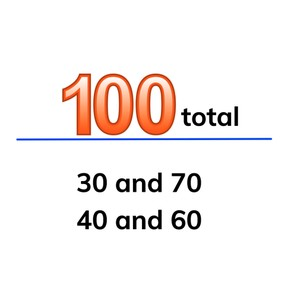Decomposing and composing 100 from round numbers

# Decomposing and composing 100 from round numbers

Decomposing and composing 100 from round numbers

No account needed.8,000 schools use Gynzy92,000 teachers use Gynzy1,600,000 students use Gynzy

## General

Students practice decomposing the number 100 from tens or fives and compose to 100.

## Relevance

It is useful to be able to do this to know possible divisions of 100. If you have saved money and want 100 dollars, it is useful to know how much more you need to save.

## Introduction

Students practice counting to 100. They skip count by 10s through the class and count to 100 together. Also practice counting forwards and backwards from randomly selected tens. Ask students if they can skip count in 5s from a number shown on the interactive whiteboard until you say stop.

## Development

Explain that you can decompose 100 into tens. If you know the decompositions of 10 well, it will be easy to decompose 100. 4 and 6 make 10, and 40 and 60 make 100. Show how they make 10 and 100 respectively. In each situation a zero is added to the end to make 100 total. Show that you can switch the numbers in a number bond. So 10 and 90 make 100 but 90 and 10 also make 100. Check that students can decompose 100 dollars by dragging tens to the children and ask students to decompose 100 in three ways. Explain that we will be composing in 10s. Then compose 100 from 25 and 75. With the 10 and 5 dollar bills, show students that they first count 5 more and then 70. Explain that 75 and 25 always make 100 and 25 and 75 also make 100. Practice with the students.

Check that students can decompose and compose 100 from tens and 25 and 75 by asking the following questions:
- How can you easily decompose 100 into tens?
- Can you name a few decompositions of 100 in tens?
- Which number goes with 25 to make 100?

## Guided Practice

Students first practice composing 100 dollars by dragging the correct amount of tens to the box. Then they decompose 100 by choosing two numbers that compose 100 and finally complete a number bond chart for 100.

## Closing

Discuss that it is important to be able to decompose 100 so you always know how much you still need. It can be useful if you deal with bills. Check that students can compose 100 with money amounts and ask students to complete the number bond chart. To close the lesson, have students play a game in pairs. The first student names a 10 smaller than 100 (or the number 100) and the second student must compose 100.

## Teaching Tip

Students who have difficulty memorizing the decompositions of 100 in tens can use MAB blocks to compose 100.

## Instruction materials

MAB blocks, number cards with tens.

### The online teaching platform for interactive whiteboards and displays in schools

• Save time building lessons

• Manage the classroom more efficiently

• Increase student engagement